# 深入学习一致性 Hash## 优秀的一致性Hash算法的特点

• 平衡性(Balance)

﻿

• 单调性(Monotonicity)

﻿

﻿

﻿

• 平滑性(Smoothness)

﻿

## 原理

### 核心概念-Hash环﻿

### 原理详解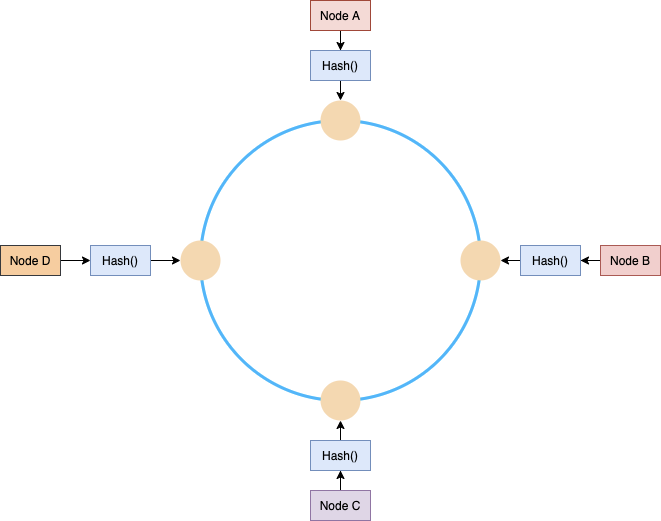﻿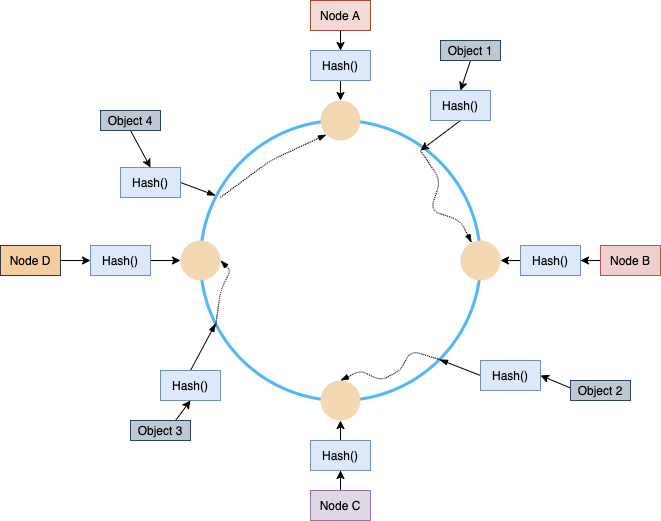﻿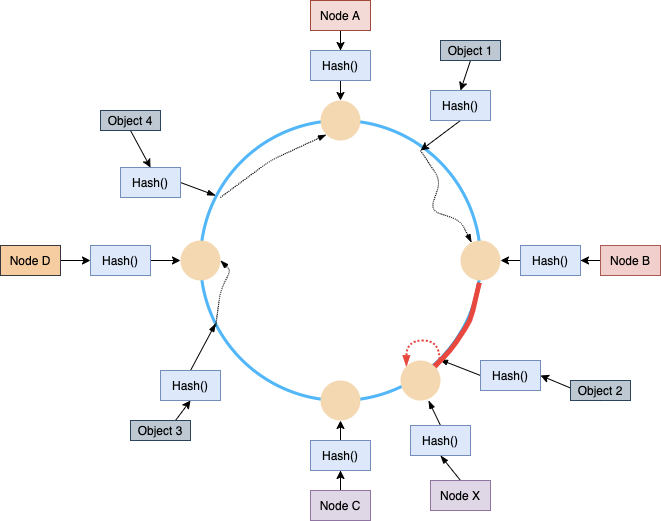﻿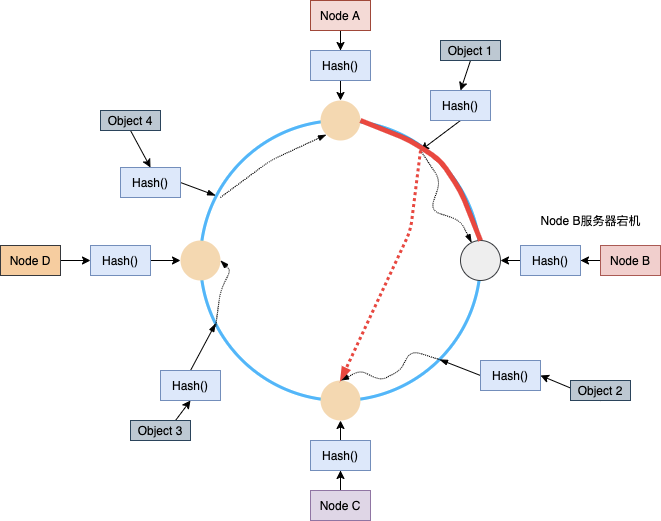﻿

﻿

### 虚拟节点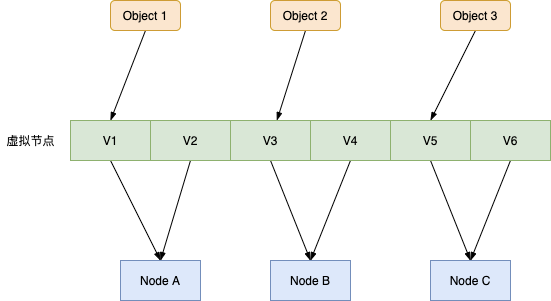﻿

## 实现

ConsistentHash.java的具体实现如下

public class ConsistentHash<T> {﻿    //Hash函数    private final HashStrategy hashStrategy;    //虚拟节点倍数    private final int virtualNodeMultiple ;    //有序Map构造Hash环    private final SortedMap<Long, T> circle = new TreeMap<>();﻿    /**     * 有参构造函数     * @param hashStrategy     * @param virtualNodeMultiple     */    public ConsistentHash(HashStrategy hashStrategy, int virtualNodeMultiple) {        this.hashStrategy = hashStrategy;        this.virtualNodeMultiple = virtualNodeMultiple;    }﻿    /**     * 添加节点     * @param node     */    public void add(T node) {        for (int i = 0; i < virtualNodeMultiple; i++) {            long key = hashStrategy.getHashCode(node.toString() + "#" + i);            circle.put(key, node);        }    }﻿    /**     * 移除节点     * @param node     */    public void remove(T node) {        for (int i = 0; i < virtualNodeMultiple; i++) {            long key = hashStrategy.getHashCode(node.toString() + "#" + i);            circle.remove(key);        }    }﻿    /**     * 获取服务器节点     * @param key     * @return     */    public T get(Object key) {        if (circle.isEmpty()) {            return null;        }        long hash = hashStrategy.getHashCode((String) key);        //如果没有对应此hash的服务器节点，获取大于等于此hash后面的服务器节点；        //如果还没有，则获取服务器节点分布圆的第一个节点        if (!circle.containsKey(hash)) {            SortedMap<Long, T> tailMap = circle.tailMap(hash);            hash = tailMap.isEmpty() ? circle.firstKey() : tailMap.firstKey();        }        return circle.get(hash);    }﻿    /**     * 求标准差     * @param map     * @return     */    public static double StandardDiviation(ConcurrentHashMap<String, LongAdder> map) {        int size = map.size();        //求和        long sum = map.values().stream().mapToLong(LongAdder::longValue).sum();        //求平均值        double dAve= sum/size;        double dVar=0;        //求方差        for(Object key:map.keySet()){            long value = map.get(key).longValue();            dVar += (value - dAve) * (value - dAve);        }        return Math.sqrt(dVar/size);    }﻿﻿    /**     * 打印hash环     * @return     */    public String printCircle() {        StringBuilder sb = new StringBuilder();        for (Map.Entry<Long, T> entry : circle.entrySet()) {            sb.append(entry.getKey()).append("=").append(entry.getValue());            sb.append("&");        }        return sb.toString().substring(0, sb.toString().length() - 1);    }}

﻿

### 通过标准差反应一致性Hash的均衡性

﻿

        //使用MD5 hash算法        HashStrategy strategy = new Md5Hash();        //预置10台服务器        String[] nodes = new String[]{"10.21.100.1","10.21.100.2","10.21.100.3","10.21.100.4","10.21.100.5","10.21.100.6","10.21.100.7","10.21.100.8","10.21.100.9","10.21.100.10"};        //预设100个虚拟节点        int virtualNodeMultiple = 100;        ConsistentHash<String> consistentHash = new ConsistentHash<>(strategy, virtualNodeMultiple);        //添加服务到和虚拟节点到Hash环上        for(String node:nodes){            consistentHash.add(node);        }        ConcurrentHashMap<String, LongAdder> map = new ConcurrentHashMap<>();        for (int i=0; i<1000000; i++){            map.computeIfAbsent(consistentHash.get(RandomString.getRandomString()), k -> new LongAdder()).increment();        }        //打印每台服务器分配到的key数量        System.out.println(map.toString());        //打印标准差结果        System.out.println(ConsistentHash.StandardDiviation(map));

﻿

﻿

-----------key分布情况------------{10.21.100.8=105590, 10.21.100.7=92469, 10.21.100.6=96057, 10.21.100.5=89047, 10.21.100.9=116228, 10.21.100.4=104149, 10.21.100.3=101953, 10.21.100.2=99218, 10.21.100.10=85484, 10.21.100.1=109805}-----------标准差------------9029.5056232332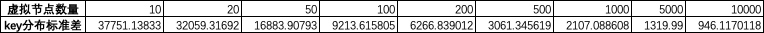﻿

### 不同Hash算法的均衡性验证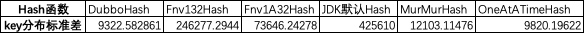﻿

﻿

public class DubboHash implements HashStrategy {﻿    @Override    public long getHashCode(String value) {        return hash(md5(value), 0);    }﻿    private long hash(byte[] digest, int number) {        return (((long) (digest[3 + number * 4] & 0xFF) << 24)                | ((long) (digest[2 + number * 4] & 0xFF) << 16)                | ((long) (digest[1 + number * 4] & 0xFF) << 8)                | (digest[number * 4] & 0xFF))                & 0xFFFFFFFFL;    }﻿    private byte[] md5(String value) {        MessageDigest md5;        try {            md5 = MessageDigest.getInstance("MD5");        } catch (NoSuchAlgorithmException e) {            throw new IllegalStateException(e.getMessage(), e);        }        md5.reset();        byte[] bytes = value.getBytes(StandardCharsets.UTF_8);        md5.update(bytes);        return md5.digest();    }}

﻿

### guava中提供的一致性hash

Guava中有一个Hashing类简单实现了一个一致性哈希的算法

﻿

//bucket是服务器节点数量，返回服务器集合的index值int bucket = Hashing.consistentHash(id, buckets)

﻿

#### 源码

﻿

public static int consistentHash(long input, int buckets) {    checkArgument(buckets > 0, "buckets must be positive: %s", buckets);    //使用LCG算法实现产生随机数    LinearCongruentialGenerator generator = new LinearCongruentialGenerator(input);    int candidate = 0;    int next;﻿    while (true) {        // 每次hash的循环中每一个的next的值总是会固定 :        next = (int) ((candidate + 1) / generator.nextDouble());﻿        if (next >= 0 && next < buckets) {            //  next范围不对，重新计算            candidate = next;        } else {            // 返回这个 candidate 值            return candidate;        }    }}﻿// LCG算法实现，可以参考 http://en.wikipedia.org/wiki/Linear_congruential_generatorprivate static final class LinearCongruentialGenerator {    private long state;﻿    public LinearCongruentialGenerator(long seed) {        this.state = seed;    }﻿    public double nextDouble() {        state = 2862933555777941757L * state + 1;        return ((double) ((int) (state >>> 33) + 1)) / (0x1.0p31);    }}

﻿

#### 均衡性效果

﻿

﻿## 评论